Stability of Prey-Predator Model with Holling type Response Function and Selective Harvesting | OMICS International
Journal of Applied & Computational Mathematics

# Stability of Prey-Predator Model with Holling type Response Function and Selective Harvesting

Jha PK1* and Ghorai S2

1School of Medical Science and Technology, Indian Institute of Technology, Kharagpur, West Bengal, India

2National Institute of Animal Welfare, Ministry of Environment Forest and Climate Change, Government of India, Faridabad, New Delhi, India

*Corresponding Author:
Senior Research Scientist
School of Medical Science and Technology
Indian Institute of Technology, Kharagpur
West Bengal, India
Tel: 03222 255 221
E-mail: [email protected]

Received Date: July 07, 2017; Accepted Date: July 31, 2017; Published Date: August 07, 2017

Citation: Jha PK, Ghorai S (2017) Stability of Prey-Predator Model with Holling type Response Function and Selective Harvesting. J Appl Computat Math 6: 358. doi: 10.4172/2168-9679.1000358

Copyright: © 2017 Jha PK, et al. This is an open-access article distributed under the terms of the Creative Commons Attribution License, which permits unrestricted use, distribution, and reproduction in any medium, provided the original author and source are credited.

Visit for more related articles at Journal of Applied & Computational Mathematics

#### Abstract

The use of mathematical models in prey predator interplay is common to solve the interdisciplinary natural problems. This paper reports analytical advancement of measuring selective harvesting activity of prey proportional to their population size and studied the stability of the model using Holling type functional response. In this paper, we analysed four prey-predatory model and considered prey and predator as a X and Y axis respectively followed by applied variational matrix and Holling I and II type response function for equilibrium and local stability measurement. Simulation experiments were carried out. Further, numerical analysis was done with help of MATLAB packages at MS window 7. Analysis of result showed prey and predator population converges asymptotically to their equilibrium values when t (time) tends to infinity and corresponding spiral phase portraits obtained. Interestingly analysis of result showed the behaviour of prey and predator with respect to time and phase portrait of the system near the equilibrium point. Above analysis indicated that application of vibrational matrix and holing type response function give better understand ability of prey predator interplay of biological forces

#### Keywords

Holling type-I and II predation function; Selective harvesting; Prey-predator; Variational matrix

#### Introduction

The prey-predator models were first modelled by Lotka-Volterra [1-5]. They used simple response function proportional to the number of predators. In prey-predator models, species normally follow different growth functions and among these [6-8], Logistic growth function is important one, which was first used by Verhulst  for human growth. Later Feller  assumed that almost every population that increases asymptotically will fit to the Logistic growth law to some degree. There also exist some other growth functions suggested by Gompertz , May . For prey-predator system, response functions in between prey and predator play an important role for the long term existence of the ecosystem. There are several types of response functions such as ratio dependent, Holling types response functions , Michaelis- Menten type, Beddington-DeAngelis [13,14] response function, etc.

The stability of ecological systems and the persistence of species within them are fundamental concerns in ecology. Mathematical models of ecological systems, reflecting these concerns, have been sued to investigate the stability of a variety of systems. For example, see [15-24]. The dynamic relationship between predator and their prey has long been and will continue to be one of the dominant themes in both ecology and mathematical ecology due to its universal existence and importance . Many excellent works have been done for the Lotka– Volterra type predator–prey system but considerable uncertainties and errors in interpretation aroused out this procedure. In ref. , Holling proposed that there exist three functional response of the predator which usually called Holling type I, Holling type II and Holling type III . He proposed the form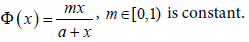as a Holling type II response function, it usually describes the uptake of substrate by the microorganisms in microbial dynamics kinetics [26,27]. If the predator is the invertebrate, it always the case. Freedmaan et al. emphasizes the application of holling type response function in preypredator interplay but also Chen and Kaung specifically mentioned the need of intercomparative analysis of holling response function for the better understanding of prey-predator interplay. Existing work does not cover comparative analysis of holling response function with vibrational matrix. For that in this paper, we have consider four preypredator models with Holling type-I and II predation function and selective harvesting of prey or predator. Keeping in view the practical implication of this study in understanding biological interplay. We elucidated the theoretical basis, equilibrium and local stability followed by verification and computation simulation for derived holling functions. This paper presents computation approach for real application of these mathematical models in biological analysis.

#### Theoretical Basis for Prey-Predator Interplay and Equilibrium Estimation

Standard theoretical model for prey-predator

Theoretical model I: Consider prey-predator system with following prey-predator model with logistic growth of prey and predation function is proportional to prey density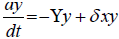(2.1)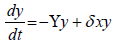(2.2)

Theoretical model II: Consider the following pre-predator system with Holling-II type predation function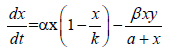(2.3)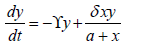(2.4)

Theoretical model III: Consider the following pre-predator system with Holling-II type predation function and constant harvesting of prey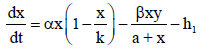(2.5)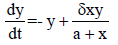(2.6)

Theoretical model IV: Consider the following pre-predator system with Holling-II type predation function and constant harvesting of predator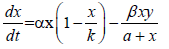(2.7)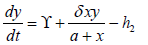(2.8)

Where, α is the intrinsic growth rate of prey, ϒ is the death rate of predator, β is the predation rate of prey, a is the half saturation constant, h1 is the constant rate of harvesting rate of prey, h2 is the constant rate of harvesting rate of predator and δ is the conversion of predator and also α, ϒ, β, a, h1, h2 and δ are all positive.

Existence and determination of equilibrium between prey-predator

As prey predator stability is based on existence of equilibrium so we determine the existence of equilibrium for above four models.

For theoretical model I: To find the equilibrium points of the system eqns. (2.1) and (2.2) we’ve,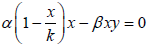(3.1)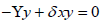(3.2)

Solving eqns. (3.1) and (3.2) we get the equilibrium points are (0, 0), (k, 0), (x*,y*).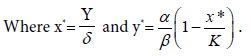For theoretical model II: Equation (2.3) and (2.4) of a particular system was used to determine the equilibrium point. We have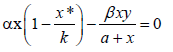(3.3)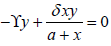(3.4)

Solving eqns. (3.3) and (3.4) we get the equilibrium points are (0, 0), (k, 0), (x*, y*).

Where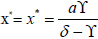and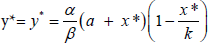and x* is positive when δ > ϒ (3.5)

For theoretical model III: Equation (2.5) and (2.6) of a particular system was used to determine the equilibrium point. We have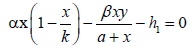(3.6)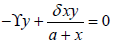(3.7)

Solving eqns. (3.6) and (3.7) we obtain the equilibrium points are (x´, 0), (x*, y*).

Where,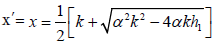and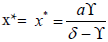and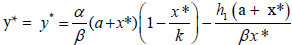x*, y* are positive as δ > ϒ and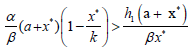(3.8)

For theoretical model IV: Equation (2.7) and (2.8) of a particular system was used to determine the equilibrium point. We have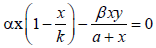(3.9)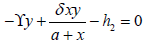(3.10)

From eqn. (3.9) we get, either x = 0 or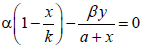(3.11)

From eqn. (3.11) we get,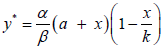.

Then from eqn. (3.10) we get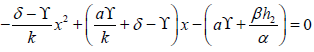There are two changes of sign. When we replace x by –x, there are no changes of sign.

So, by Descarte’s rule of sign there are exactly two positive roots. Say, one root be x*.

Therefore, the equilibrium point is (x*, y*).

#### Experimental Setting and Verification for Local Stability

Local stability of these four models is discussed with variational matrix.

For theoretical model I: The variational matrix of the system eqns. (2.1) and (2.2) is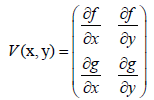Where,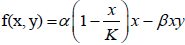g(x, y) = −Υy +δxy.

Therefore,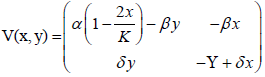Now discuss the stability near the (0, 0)

At the point (0, 0),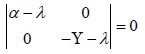The characteristic equation of the corresponding variational matrix is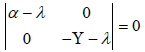Therefore, λ =α > 0

λ = −γ

Eigen values are real distinct and opposite sign so the equilibrium pint is a saddle point and therefore the system is unstable.

At the point (k, 0),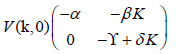The characteristic equation corresponding variational matrix is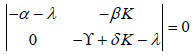Therefore, λ = −α < 0

λ = −(γδ K) < 0 when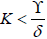and λ = −(γδ K) > 0 when.

Therefore, the roots are real distinct and negative therefore the equilibrium point is a node. So the system is asymptotically stable if.

Now at the point (x*, y*),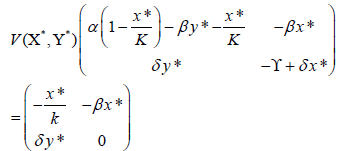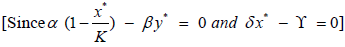The characteristic equation corresponding varitional matrix is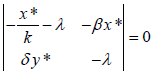Or,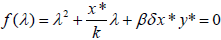Now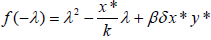There are two changes of sign. So, by Descarte’s rule of sign there are two negative roots.

Therefore, the system have stable node.

For theoretical model II: The variational matrix of the system (2.3) and (2.4) is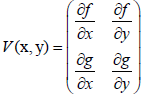Where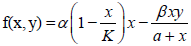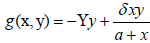Therefore,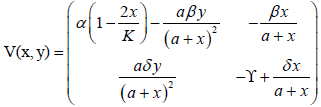At the point (0, 0),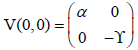So, the characteristic equation is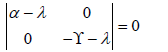Therefore, λ = α > 0 and λ = − ϒ < 0

Hence, the system is unstable.

At the point (k, 0),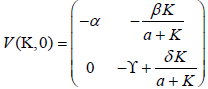The characteristic equation is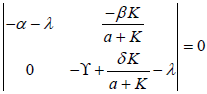Therefore, λ = −α < 0 and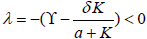when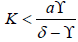.

Therefore, the roots are real distinct and negative therefore the equilibrium point is a node. So the system is asymptotically stable if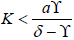.

Now, at the point (x*, y*),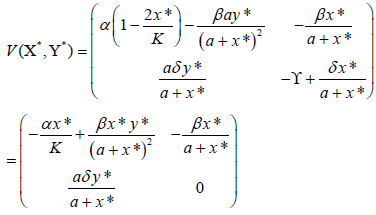The characteristic equation is,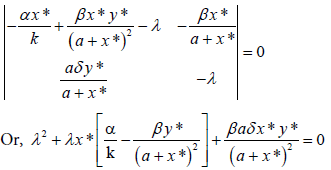If we replace λ by –λ, then there are two changes of sign when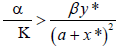.

So, by Descarte’s rule of sign there are two negative roots when.

Hence, therefore, the roots are real distinct and negative or complex with negative real part therefore the equilibrium point is a node. So the system is asymptotically stable when. (A)

For theoretical model III: The vibrational matrix of the system eqns. (2.5) and (2.6) is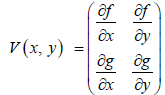Where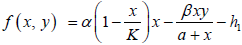,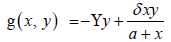Therefore,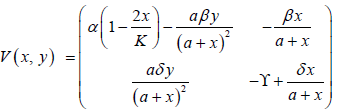At the point (x´, 0),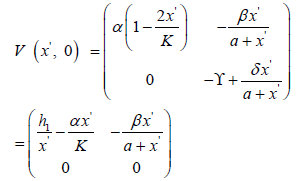The characteristic equation of the corresponding variational matrix is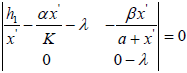Or,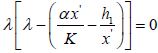Therefore, either λ = 0 or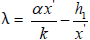So, the system is not stable.

Now, at the point (x*, y*),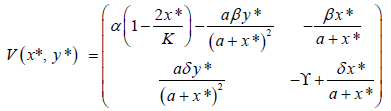The characteristic equation of the corresponding variational matrix is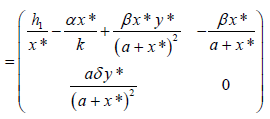Or,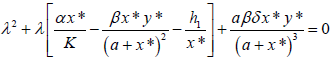If we replace λ by –λ, then there are two changes of sign when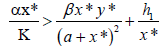.

So, by Descarte’s rule of sign there are two negative roots when.

Hence, therefore, the roots are real distinct and negative or complex with negative real part therefore the equilibrium point is a node.

So the system is asymptotically stable ifFor Theoretical model III: The variational matrix of the system (2.7) and (2.8) is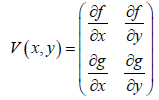Where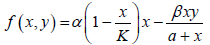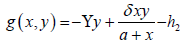Therefore,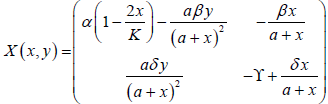Now, at the point (x*, y*),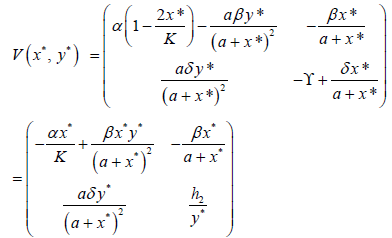The characteristic equation of the corresponding variational matrix is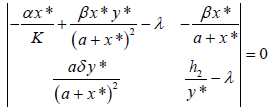Or,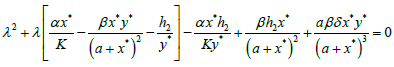If we replace λ by –λ, then there are two changes of sign when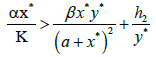.

So, by Descarte’s rule of sign there are two negative roots when.

Hence, therefore, the roots are real distinct and negative or complex with negative real part therefore the equilibrium point is a node. So the system is asymptotically stable when#### Results and Discussion

It was observed that the bifurcation of the theoretical model- II with respect to the parameter a half saturation constant. The roots of characteristic equation are real distinct negative or complex with negative real part if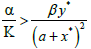(From A). So the system is asymptotically stablei.e.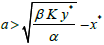.

For theoretical model-III the system is asymptotically stable if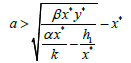For theoretical model-IV the system is asymptotically stable if.

Numerical example: In this section numerical simulation is present to illustrate the behaviour of prey and predator with respect to time and phase portrait of the system near the equilibrium point which results obtained in previous sections. Now choose the values of parameters for different model.

For Model-I: α= 1.8;K=20; β=0.03; δ=0.05; γ=0.3; (Figure 1A).

Figure 1: A) Prey and predator population asymptotically converge to the equilibrium state value; B) Phase portrait of the system showing that (x*, y*) is locally asymptotically stable.

For Model-2: α=1.8; K=10; β=0.6; δ=0.9;γ=0.1; a=9; for these values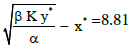and the condition of stability with respect to the parameter a is satisfied as 9>8.81 (Figure 1B).

For Model-IV α=1.8; K=10; β=0.6; δ=0.9; γ =0.1; a= 8; for these values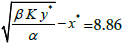and the condition of stability with respect to the parameter a is not satisfied as 8 < 8.81( 8 is not greater than 8.86) (Figure 2A and 2B).

Figure 2: A) Prey and predator population asymptotically converge to the equilibrium state value; B) Phase portrait of the system showing that (x*, y*) is locally asymptotically stable.

For Model-IV:

α=1.8;K=10; β=0.5; δ=0.6; γ =0.1; α = 10 h1=10; (Figures 3 and 4).

Numerical simulation of Model-4 is similar to Model-3 (Table 1).

Model at (0,0) At (x,0) at (x,y) Phase portrait
Model-1 Unstable Asymptotically stable Stable Figure 1A and 1B
Model-2 Unstable Asymptotically stable Asymptotically stable if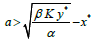Figures 2A and 2B
Model-3 - Unstable Asymptotically stable if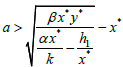Figures 3 and 4
Model-4 - - Asymptotically stable if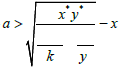Same as model-3

Table 1: Nature of equilibria of four models.

#### Discussion, Conclusion and Future Extension:

Four mathematical prey-predatory population

In this paper, four mathematical prey-predator population models are considered and analysed. In these models we consider the Logistic law of growth of prey. We consider Holling-I type predator response function in model-1 and in models-2,3 and 4 Holling – II type predator response function. Also consider selective harvesting of prey and predator. In model 3, consider constant prey harvesting only and constant predator harvesting in modes l-4. The conditions of existence of several equilibrium points have been examined. Local stability of the equilibrium points are discussed by variational matrix and also derive the conditions of asymptotical stability of equilibrium points. Discuss the bifurcation of the model-2 with respect to the parameter a (half saturation constant) and also derive the condition of existence of bifurcation. Numerical simulation is done by MATLAB software. The behaviour of prey and predator population with respect to time and phase portrait of the system near the equilibrium point is presented. It is observed that with the given values of parameters the prey and predator population converges asymptotically to their equilibrium values when t (time) tends to infinity and corresponding spiral phase portraits are obtained which are presented. Bifurcation of the model-2 is discussed for two values of a (half saturation constant) (i.e., a=9 and a=8). For a=9, the condition of stability is satisfied i.e. 9>8.81 and for a=8, the condition of stability is not satisfied i.e., 8<8.81 and oscillatory behaviour of the prey and predator population and periodic phase portrait are presented.

These models can be further extended by other type of response functions such as Beddington-De Angelis sigmoid response function etc., Selective harvesting by combined harvesting and also constant rate of harvesting by variable rate of harvesting. The prey-predator model can be extended by three species food chain model i.e. prey, predator and super predator.

#### References

Select your language of interest to view the total content in your interested language

### Article Usage

• Total views: 2458
• [From(publication date):
September-2017 - Dec 07, 2019]
• Breakdown by view type
• HTML page views : 2149Can't read the image? click here to refresh